# Appendix 1

The derivation of the Gauss-Markov theorem depended upon the matrix identity,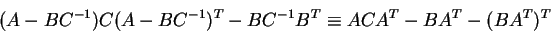(1)

This identity is not very intuitive and so we will provide the proof here. This proof depends upon one assumption being true, that C = CT.

Let us define,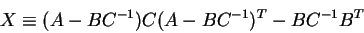(2)

and,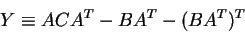(3)

If we can establish that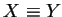, then we have our proof.

We start by expanding X,

 X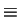(A - B C-1) C (A - B C-1)T - B C-1 BT = (A - B C-1) C (AT - C-TBT) - B C-1 BT (4)

and since we have assumed that C = CT, then C-1 = C-T, so
 X = (A - B C-1) C (AT - C-1BT) - B C-1 BT = (A C - B ) (AT - C-1BT) - B C-1 BT = ACAT - BAT - ABT + BC-1BT - BC-1BT =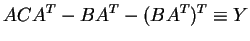(5)

Q.E.D

Skip Carter
1999-12-09